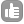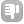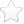0Hi All, I've been trying to get a clear answer to this question for a while now, but can't seem to get a definitive answer. My approach is following the method described in "Practical NMR for organic chemists" by John Hollerton and Steve Richards. I don't take any issue with this method, by only problem is that it gives a WILDLY different answer to the one I obtain when I do NMR quantification in TopSpin... this is a big problem, since often we are interested in the % of unwanted molecules in our samples. Let me give you a simplified example of my problem: Say I have a proton NMR spectrum infront of me. It contains my compound, plus a nominal amount of residual solvent such as dichloromethane. If I integrate a single proton first, and set the integral of this to 1.00, and then integrate the dichloromethane peak afterwards, and this comes out as 0.02, then I would be correct ( I think) in saying that there is 2mol% of dichloromethane relative to my sample, since integrals are directly proportional to concentration (so for every 100 mol of compound, I'd have 2 mol of dichloromethane)... so far so good, right? In Hollerton's book, he then says this can be converted to a relative % weight by multiplying this molar percentage by (Mwt Residual solvent / Mwt of compound). Again, so far so good. The problem is that, as I say, this gives a very different answer to the one obtained in topspin, and I would value anyones understanding of why this may be... Do I need to scale my answer by some factor? As a side question: say I added an exact quantity of some other reference, say 0.1 mL of benzene, would I easily be able to calculate the exact weight of my compound, knowing the relative integrals, and molecular weights?? Kind regards, and thankyou in advance!! asked Oct 30 '13 at 16:330In relation to your example, dichloromethane has 2 protons per molecule so first you have to divide the integral by 2. then mol% = 0.01 / (1.0 + 0.01) = 0.99mol% ie you divide by the sum of all the components. This doesn't answer your question fully but I hope it is helpful. To calculate wt% you must sum the products of the integral and MW ie, 0.01 * MW(DCM) / (0.01 * MW(DCM) + 1.0*MW(Compound1) + ......) answered Nov 11 '13 at 15:500Firstly may I congratulate you on an excellent book choice ;-) Victoria is quite right that you need to normalise the number of protons in your compounds of interest. Effectively you must be comparing the same things (one proton with one proton). There are some other things to watch out for which are covered in the book. If you are looking at solvents then there is a good chance that their relaxation times are very different to your main molecule. To get a decent answer you will need a longer relaxation delay. If you are using a 30 degree pulse (zg30 in Bruker terms) then you could probably get away with a total recycle time (aq + d1) of 15 seconds. If you want to be sure then you would need to measure the T1s (or leave a very long relaxation delay). If you have everything set up correctly, your main source of error is the measurement of the integral. NMR is great for quantification. If you want an approximate answer then it is really quick and simple. The more accuracy and precision you need, the more complex the experiment becomes! answered Dec 09 '13 at 09:37toggle preview

Tags:

×26
×2

Asked: Oct 30 '13 at 16:33

Seen: 24,038 times

Last updated: Dec 09 '13 at 09:37